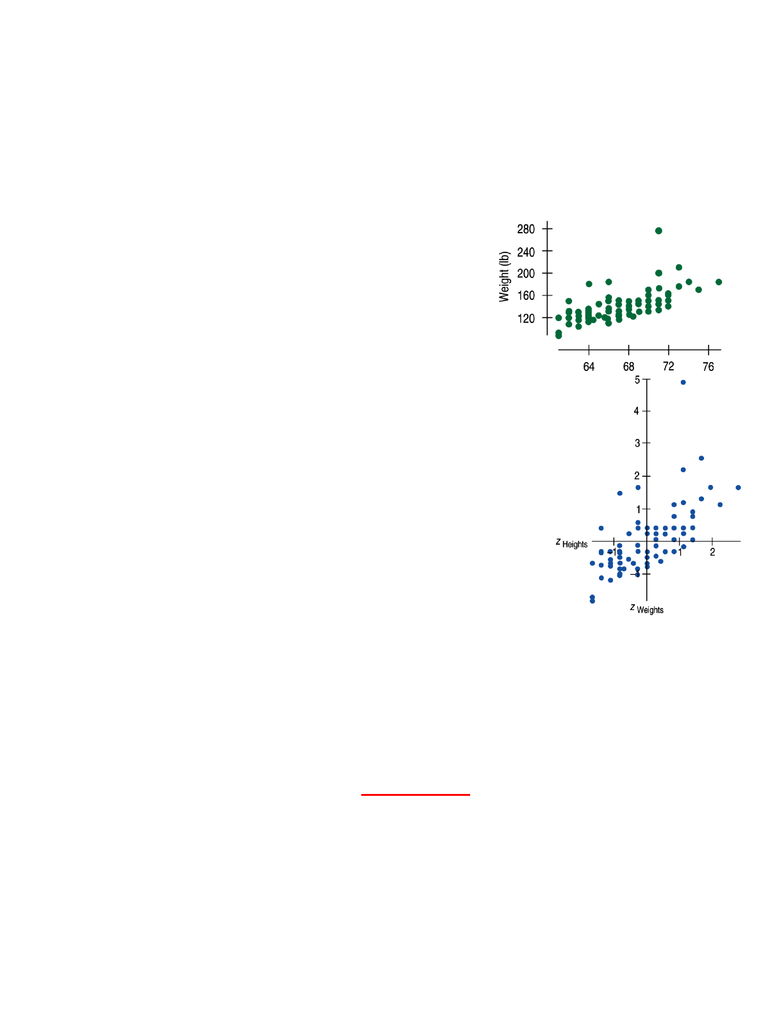Textbook Notes (280,000)
CA (160,000)
U of A (2,000)
STAT (100)
STAT141 (10)
Chapter

# STAT141 Chapter Notes -Confounding, Scatter Plot, Dependent And Independent Variables

Department
Statistics
Course Code
STAT141
Professor
Peter Hooper

This preview shows pages 1-3. to view the full 15 pages of the document.Statistics Part Two – Exploring Relationships
Between Two Variables
Chapter Seven – Scatterplots, Association and
Correlation
Scatterplots
Scatterplots may be the most common and most effective display for
data
In a scatterplot, you can see patterns, trends, relationships, and
even the occasional extraordinary value sitting apart from the
others
Scatterplots are the best way to start observing the relationship and
the ideal way to picture associations between two quantitative
variables
Roles for Variables
It is important to determine which of the two quantitative variables
goes on the x-axis and which on the y-axis
This determination is made based on the roles played by the variables
When the roles are clear, the explanatory or predictor variable goes on
the x-axis and the response variable goes on the y-axis
The roles that we choose for variables are more about how we think
Just placing a variable on the x-axis doesn’t necessarily mean that it
explains or predicts anything. And the variable on the y-axis may not
respond to it in any way
More on Scatterplots
When looking at scatterplots, we will look for direction, form, strength,
and unusual features.
Direction:
A pattern that runs from the upper left to the lower right is said
to have negative direction
A trend running the other way is said to have positive direction
Form:
If there is a straight line (linear) relationship, it will appear as a
cloud or swarm of points stretched out in a generally consistent,
straight form
If the relationship isn’t straight, but curves gently (exponential
growth for example), while still increasing or decreasing
steadily, we can often find ways to make it more nearly straight
If the relationship curves sharply however, the methods will not
work
Strength:
At one extreme, the points appear to follow a single stream
(whether straight, curved, or bending all over)
At the other extreme, the points appear as a vague cloud with
no discernable trend or pattern

Only pages 1-3 are available for preview. Some parts have been intentionally blurred.Unusual Features:
Look for the unexpected
Often the most interesting thing to see in a scatterplot is the
thing you never thought to look for
One example of such a surprise is an outlier standing away from
the overall pattern of the scatterplot
Clusters or subgroups should also raise questions
Correlation: Quantifying the Strength of Linear
Association
Data collected from students in stats classes
included their heights and weights
Here is a positive association and a fairly
straight form with one high outlier.
So how strong is the association between
weight and height of stats students?
If we had to put a number on the strength,
we would not want it to depend on the units
we used because no matter the units, the pattern is
the same
So since units do not matter, why not remove them?
We could standardize both variables and write the
coordinates of a point as (zx, zy)
Here is a scatterplot of the standardized weights and
heights
Note that the underlying linear pattern seems
steeper in the standardized plot than in the
original
That’s because we made the scales of the axis
the same
Equal scaling gives a neutral way of drawing
the scatterplot and a fairer impression of the
strength of association
Some points strengthen the impression of a positive association (along
linear line), others weaken the positive (outliers) and some don’t vote
either way (z-scores of zero)
The correlation coefficient (r) gives us a numerical measurement of the
strength of the linear relationship between the explanatory and the
response variables
1
x y
z z
rn
=
(So the formula means multiply each zx by zy, add up all those values,
then divide by the number of data minus one)
Correlation Conditions

Only pages 1-3 are available for preview. Some parts have been intentionally blurred.Correlation measures the strength of the linear association between
two quantitative variables
Before you use correlation, you must check several conditions
Quantitative Variables Condition
Correlation applies only to quantitative variables
Don’t apply correlation to categorical data masquerading
as quantitative
Check that you the variables’ units and what they
measure
Straight Enough Condition
You can calculate a correlation coefficient for any pair of
variables
But correlation measures the strength only of the linear
association, and will be misleading if the relationship is
not linear
Outlier Condition
Outliers can distort the correlation dramatically
An outlier can make an otherwise small correlation look
big or hide a large correlation
It can even give an otherwise positive association a
negative correlation coefficient (and vice versa)
When you see an outlier, it’s often a good idea to report
the correlations with and without that point
Correlation Properties
The sign of a correlation coefficient gives the direction of the
association
Correlation is always between -1 and +1
Correlation can be exactly equal to -1 or +1, but these values
are unusual in real data because they mean that all the data
points fall exactly on a single straight line
A correlation near zero corresponds to a weak linear association
Correlation treats x and y symmetrically:
The correlation of x with y is the same as the correlation of y
with x
Correlation has no units
Correlation is not affected by changes in the center or scale of either
variable
Correlation depends only on the z-scores, and they are
unaffected by changes in center or scale
Correlation DOES NOT EQUAL Causation
Whenever we have a strong correlation, it is tempting to explain it by
imagining that the predictor variable has caused the response to help
Scatterplots and correlation coefficients never prove causation
A hidden variable that stands behind a relationship and determines it
by simultaneously affecting the other two variables is called a lurking
variable
Also: